# matlab中if语句多个_MATLAB入门教程 | 003基础知识1. MATLAB概述

(1)在欧美各高等学校, Matlab成为线性代数、自动控制理论、数字信号处理、时间序列分析、动态系统仿真、图像处理等诸多课程的基本教学工具，成为本科生、硕士生和博士生的必须掌握的基本技能。

(2)在设计研究单位和工业部门, Matlab已被广泛地用于研究和解决各种具体的工程问题。

(3)可以预见，Matlab将在我国科学研究和工程应用中发挥越来越大的作用。

2. MATLAB特点

• 数值运算优势

• 符号运算优势

• 强大的2D、3D数据可视化功能

• MATLAB以数组为基本计算单元

• 具有大量的算法优化功能函数

• 编程易、效率高

• 语言及书写形式非常接近于常规数学书写形式

• 完备的帮助系统，易学易用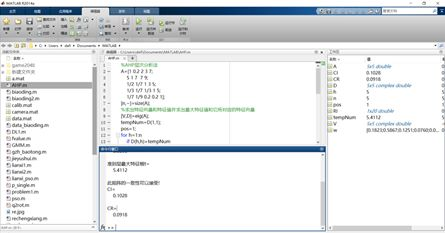MATLAB主页面

3. 命令窗口的使用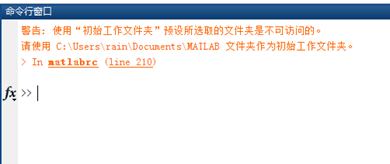MATLAB命令窗口

“>>”与光标的闪烁一起说明系统就绪，等待输入；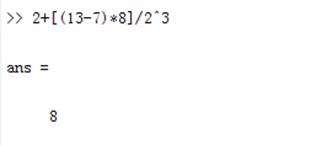MATLAB所用的运算符如(加减乘除等)是各种计算程序中常见的；

4. 创建M文件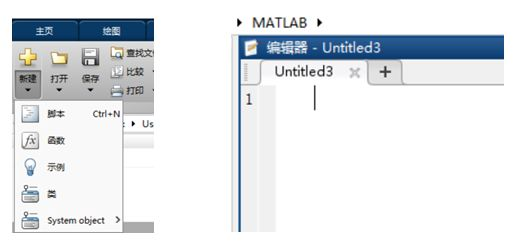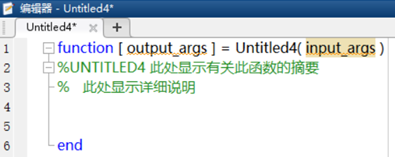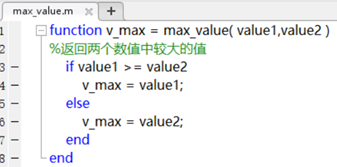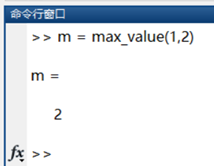5. 目录和文件管理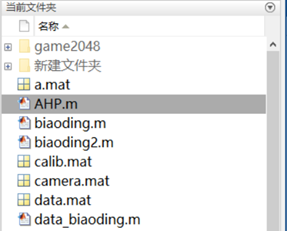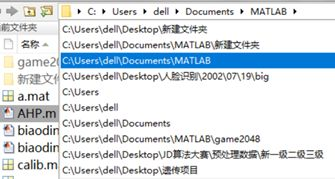6. 搜索路径管理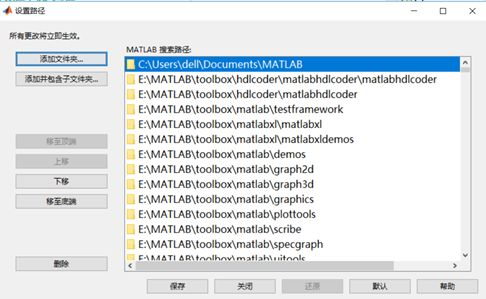1. MATLAB简单计算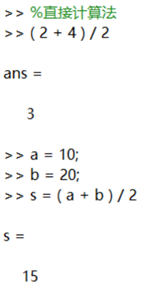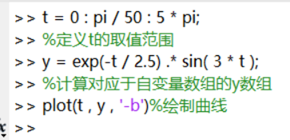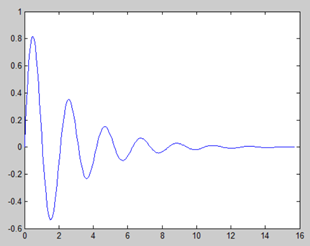2. 基本运算符号

Matlab进行数学处理时的优先级顺序和通常进行数学处理的优先级顺序一致。指数优先；乘、除次之；括号改变优先级，表达式从左到右依次运算。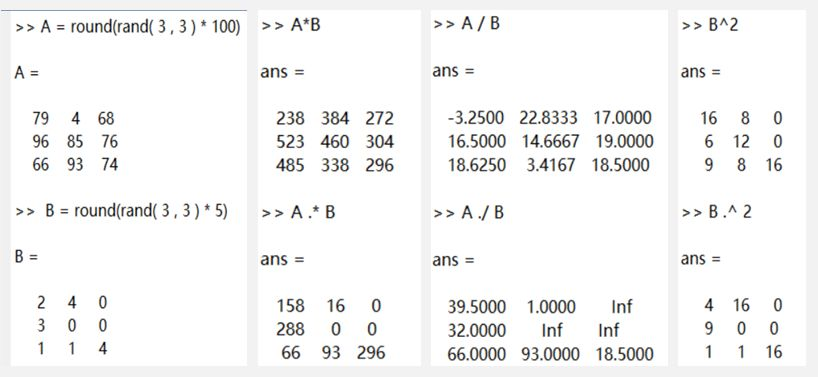3. 数值、变量及表达式

MATLAB的数只采用习惯的十进制表示，可以带小数点和负号，缺省了双精度浮点型数据。

1、变量名、函数名对字母区分大小写；

2、变量名可以包含英文字母、下划线和数字，但必须以英文字母开头；

3、变量名最多可以包含63个字符。

MATLAB预定义变量：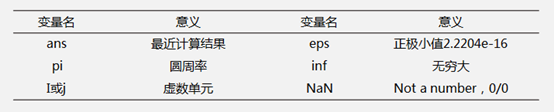4. 数组

(1)数组生成

1、  直接输入法：通过空格、逗号和分号来分隔数组元素，在数组中输入任意元素，生成一维数组。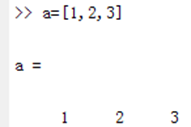2、步长生成法：x = a : step :b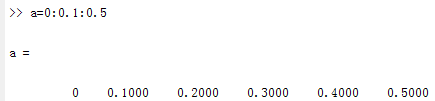3、等间距线型生成法：x =linspace(a,b,n)，在a到b区间内生成n个采样点数据。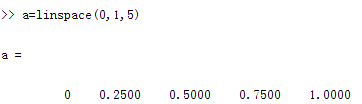1、  直接输入法，同行之间元素用逗号或空格分隔，不同行之间用分号分隔。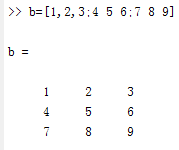2、  调用内置函数，如zeros,ones,rand等。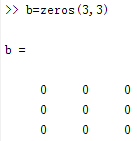3、低维数组转换，通过reshape，cat等函数将低维数组转换成高维数组。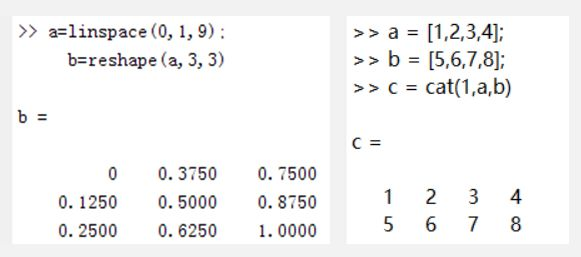(2)数组寻访

a=[1 2 3;4 5 6;7 8 9];

a(2,3) a(2,:)  a(:,1)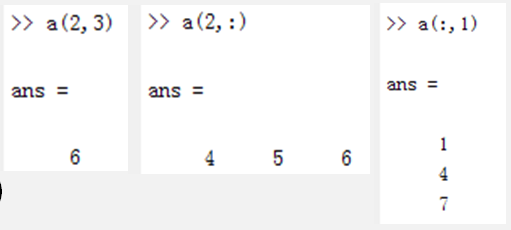a(1,1:2) a(2,1:end-1)   a(1,:)=[]  a([1,3],[2,3])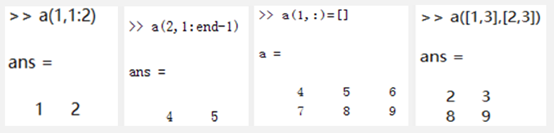[As,idx] = sort(A)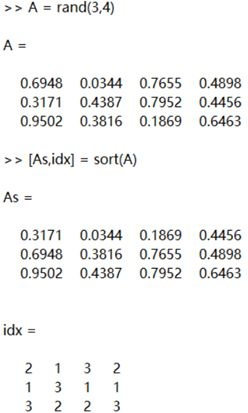[As_r,idx_r] = sortrows(A)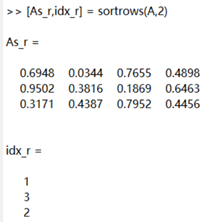[i,j] = find(A>0.5)

k = find(A>0.5,1)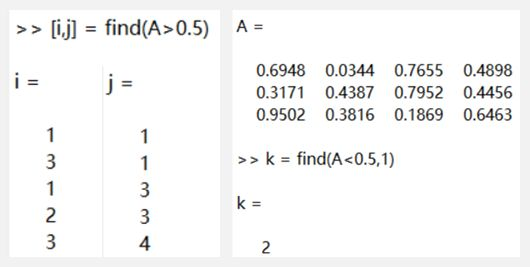[mx,rx] = max(A)

[mn,rx] = min(A)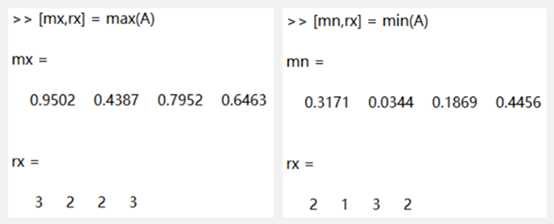1. 流程控制

(1)for循环结构

for循环结构中，需要设定一定的循环条件，Matlab根据设定的循环次数执行循环体内的命令。

for x = array

commands

end

%for循环结构示例

for n = 10 : -1 : 1

x(n) = sin(n * pi / 5);

end

x

array = ceil(rand(1,10) * 10);

for n = array

x(n) = sin(n * pi / 5);

end

x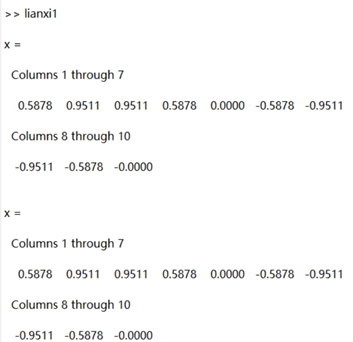(2)while循环结构

while循环结构对循环体进行无限次的循环运算，直到循环体满足循环结束条件，或达到一定的循环次数后终止。

while expression

commands

end

%while循环结构示例

%求浮点数eps的精度

EPS = 1;

num = 0;

while (EPS + 1 ) > 1

EPS = EPS / 2;

num = num + 1;

end

num

EPS = EPS * 2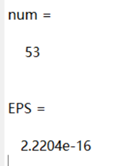(3) if判断结构

if判断结构

根据某一给定的条件，来进行判断以执行不同的命令。

if-else-end判断结构

处理判断条件为true或false时，分别执行不同的命令行。

if expression

commands

end

if expression

commands1

else

commands2

end

%if判断结构示例

%求一元二次方程a*x^2 + b*x +c = 0的根

a = 2; b = 3; c = 5;

delta = b^2 - 4*a*c;

if delta > 0

x1 = (-b+sqrt(delta))/(2*a)

x2 = (-b-sqrt(delta))/(2*a)

elseif delta == 0

x1 = (-b+sqrt(delta))/(2*a)

else

real_a = -b/(2*a);

imag_b = sqrt(abs(delta)) / (2*a);

x1 = real_a - imag_b*i

x2 = real_a + imag_b*i

end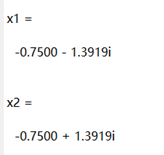2. 控制命令

continue命令主要用在循环语句中，提前结束循环体的本次操作，将continue直接放在循环控制体内，以配合if判断语句使用。

break命令和continue命令一样都用在循环结构中，当执行break命令时，程序跳出循环结构，转移到循环之外的下一条语句。

continue命令使程序跳转到循环的end语句处，结束一次循环，而break命令使程序跳出break所在的循环体。

%continue break控制语句示例

%计算浮点数eps的精度

EPS = 1;

for n = 1:1000

EPS = EPS / 2;

if (1 + EPS ) >1

continue

end

EPS = EPS * 2;

break;

end

EPS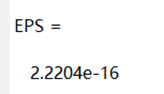3. 程序向量化概念

%循环结构

for i = 1:100

s1(i) = i^2;

end

%向量化处理

s2 = [1:100].^2;

%循环结构

tic

num_max = 1000000;

for i = 1:num_max

s1(i) = i^2;

end

toc

%向量化处理

tic

s2 = [1:num_max].^2;

toc

4. 逻辑数组和向量化

Matlab除了基本的数值数据类型和字符串之外，逻辑数据也是一种数据类型。逻辑数据可以通过关系和逻辑表达式来创建，也可以通过logical命令来创建逻辑数组。

%循环结构

tic

num_max = 1000000;

for i = 1:num_max

if i < 500000

s1(i) = i^2;

else

s1(i) = i;

end

end

toc

%向量化处理

tic

a = 1:num_max;

s2 = a(a<500000).^2;

toc

往 期 推 荐

Matlab进阶教程 | 提取黑白照片亮度制作彩图

Matlab 入门教程|长投复利收益问题

Matlab入门教程 | 001 球的体积问题08-0244512-01183
01-185万+
01-134004
11-21280
11-2239
04-1310万+
08-23
04-165384
11-011935
07-085160
11-2751
11-27936
04-107984
12-031万+
11-23点击重新获取扫码支付余额充值